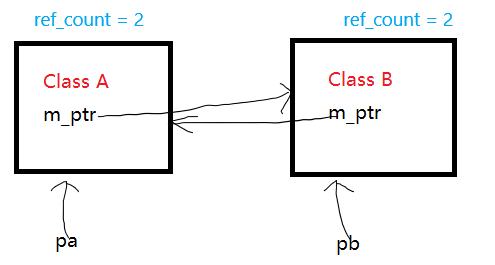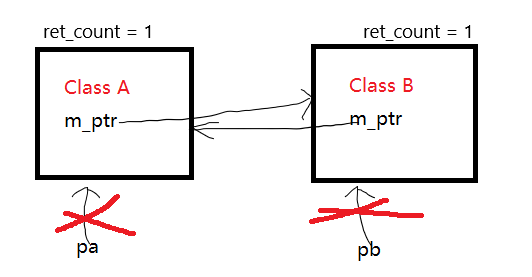$C++ 11$ 中提供了四个智能指针，分别为 auto_ptr、unique_ptr、shared_ptr、weak_ptr，第一个被舍弃暂时不谈，第二个没啥说的，本文主要介绍 shared_ptr、weak_ptr，在使用过程中的问题 (在面试中经常看见)

首先，本文默认读者了解智能指针的相关用法及含义。

### 循环引用

循环引用的发生类似于死锁。使用智能指针会帮助程序员减少内存泄露的可能性，但是如果智能指针的使用方式不对，一样会造成内存泄漏，比价典型的情况是如下的循环引用

class B; // 前置声明
class A {
public:
shared_ptr<B> ptr;
};

class B {
public:
shared_ptr<A> ptr;
};

void test(){
shared_ptr<A> pa(new A());
shared_ptr<B> pb(new B());
pa -> ptr = pb;
pb -> ptr = pa;
}上图中， $Class A$ 和 $Class B$ 的对象各自被两个智能指针管理，$pa$ 和 $pb$ 的引用次数都是 $2$，为什么？对 $Class A$ 分析，被同时被 $pa$ 与 $Class B$ 中的 $ptr$ 共同拥有，所以其中的计数器为 $2$。

在当前情况下，当 $test()$ 函数结束时，$pa$ 和 $pb$ 的析构函数被调用，但是 $class A$ 对象和 $class B$ 对象仍然被一个智能指针管理，他们的引用计数变成 $1$，导致两个对象的内存无法被正常析构释放，产生内存泄漏，如下图所示。在上述情况产生了一个闭环，$A$ 对象想要释放干净就必须要先把 $B$ 对象里面的 $A$ 指针消除，$B$ 对象也同理。所以解决的方法就是打破这个闭环，让其中一个计数器变成 $1$，即可。所以 $C++ 11$ 引入了 weak_ptr 弱指针，来解决这个问题。它不会对原来的智能指针引用次数进行操作，而是对单独的一个计数器进行操作。把 $class A$ 或者 $class B$ 中的 shared_ptr 改成 weak_ptr 即可，由于weak_ptr 不会增加 shared_ptr 的引用计数，所以 $A$ 对象和 $B$ 对象中有一个的引用计数为$1$，在 $pa$ 和 $pb$ 析构时，会正确地释放掉内存。

### 为什么能解决

跟踪了一下 shared_ptrweak_ptr 的代码发现了它们均是继承于一个 _Ptr_base 的类。shared_ptrweak_ptr 都没有单独的成员变量，而是继承了 _Ptr_base 中的 _Ptr_Rep， 前一个代表内部指针，后一个代表引用计数器。当智能指针被使用的时，会将内部的 _Uses_Weaks 同时设置为 1，当添加 shared_ptr 时，会让内部的 _Use++，使用 weak_ptr 时会让 _Weaks++，所以使用弱指针不会影响本身的引用计数器。下面是简单实现的一个智能指针，只实现了智能指针的思想。

#include <iostream>
using namespace std;

class CRefCount{	//计数器类
public:
CRefCount() {	//产生一个智能指针就把里面的两个计数器复制为 1
m_nUsedCount = 1;
m_nWeakCount = 1;
}

void incUsed() { m_nUsedCount++; }	//对与强指针的使用会改变自身的引用计数器

int decUsed() {	//当一个强指针被析构，自身引用次数减少
m_nUsedCount--;
return m_nUsedCount;
}

void incWeak() { m_nWeakCount++; }	//对于弱指针的单独计数

int decWeak() {	//对于弱指针消失的操作
m_nWeakCount--;
return m_nWeakCount;
}
private:
int m_nUsedCount;	//记录强指针引用次数
int m_nWeakCount;	//记录弱指针引用次数
};

template<typename T>
class CMySmartPtrBase {	//智能指针的基类
public:
CMySmartPtrBase() = default;
~CMySmartPtrBase() = default;

void destroy() { //删除内部的指针
delete m_Ptr;
}

void release(){
if(m_pRef != nullptr && m_pRef->decWeak() == 0) {    //删除内部的计数器
delete m_pRef;
}
}

protected:
T* m_Ptr;	//自身的指针
CRefCount* m_pRef;	//引用计数器
};

template<typename T>
class CMyWeakPtr;	//提前声明弱指针

template<typename T>
class CStrongPtr : public CMySmartPtrBase<T> {	//强指针
friend class CMyWeakPtr<T>;	//将弱指针设为自己的友元类
public:
CStrongPtr(){
this->m_Ptr = nullptr;
this->m_pRef = nullptr;
}

explicit CStrongPtr(T* p) {	//有参构造
this->m_Ptr = p;
this->m_pRef = new CRefCount;
}

CStrongPtr(CStrongPtr<T>& obj){	//拷贝构造
this->m_Ptr = obj.m_Ptr;	//两个指针指向同一片内存
obj.m_pRef->incUsed();	//调用被拷贝的对象增加他的引用次数
this->m_pRef = obj.m_pRef;
}

CStrongPtr<T>& operator=(CStrongPtr<T>& obj) {	//赋值构造
if(this->m_pRef != nullptr && this->m_pRef->decUsed() == 0){	//如果该指针的引用次数已经为0，释放该指针的所有东西。
this->destroy();
this->release();
}

this->m_Ptr = obj.m_Ptr;
obj.m_pRef->incUsed();
this->m_pRef = obj.m_pRef;

return *this;
}

T& operator*(){
return *this->m_Ptr;
}

T& operator->(){
return *this->m_Ptr;
}

T* get(){
return *this->m_Ptr;
}

~CStrongPtr(){
if(this->m_Ptr != nullptr && this->m_pRef->decUsed() == 0) {	//如果引用次数为零直接释放
this->destroy();
this->release();
}
}
};

template<typename T>
class CMyWeakPtr:public CMySmartPtrBase<T>{	//弱指针同理
friend class CStrongPtr<T>;
public:
CMyWeakPtr(){
this->m_Ptr = nullptr;
this->m_pRef = nullptr;
}

CMyWeakPtr(CStrongPtr<T>& obj){
this->m_Ptr = obj.m_Ptr;
obj.m_pRef->incWeak();
this->m_pRef = obj.m_pRef;
}

CMyWeakPtr<T>& operator=(const CStrongPtr<T>& obj){
this->release();

this->m_Ptr = obj.m_Ptr;
obj.m_pRef->incWeak();
this->m_pRef = obj.m_pRef;
}

~CMyWeakPtr() {
this->release();
}

CStrongPtr<T>& lock(){
if(this->m_pRef == nullptr)
return CStrongPtr<T>();

return CStrongPtr<T>(*this);
}

bool IsExpried(){
if(this->m_pRef == nullptr)
return true;
return this->m_pRef->getUsed() == 0;
}
};

class CSon;

class CTest{
public:
void set(CStrongPtr<CSon> p2) {
this->m_p1 = p2;
}
CStrongPtr<CSon> m_p1;
};

class CSon{
public:
void set(CStrongPtr<CTest> p2) {
this->m_p1 = p2;
}
CMyWeakPtr<CTest> m_p1;
};

void foo(){
CTest* father = new CTest();
CSon* son = new CSon();

CStrongPtr<CTest> ptrFather(father);
CStrongPtr<CSon> ptrSon(son);

father->set(ptrSon);
son->set(ptrFather);
}

int main(){
foo();
system("pause");
return 0;
}